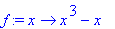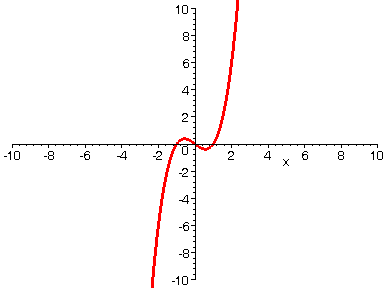VERTICAL SHIFT

If you take a function y = f(x) and replace it by y = f(x) + k where k > 0, then the net result graphically is that the graph of f(x) is shifted vertically upward. Similarly, k < 0 would produce a downward shift. Below we start with a polynomial function of the form y = f(x) + k, and the animation shows the effect on the graph as we let k increase from 0 to 10.

> with(plots):

> f:=x->x^3-1*x;> A := seq(plot(f(x)+i, x=-10..10, numpoints=500, thickness=3), i=0..10):

> display(A, insequence=true, view=[-10..10, -10..10]);>